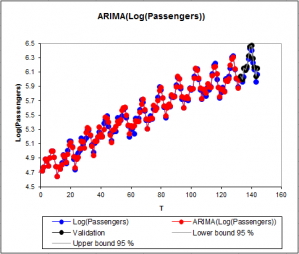# ARIMA

ARIMA models describe phenomena that evolve through time and predict future values. Run them in Excel using the XLSTAT add-on statistical software.XLSTAT offers a wide selection of ARIMA models such as ARMA (Autoregressive Moving Average), ARIMA (Autoregressive Integrated Moving Average) or SARIMA (Seasonal Autoregressive Integrated Moving Average).

## What are ARIMA models

The models of the ARIMA family allow to represent in a synthetic way phenomena that vary with time, and to predict future values with a confidence interval around the predictions.

The mathematical writing of the ARIMA models differs from one author to the other. The differences concern most of the time the sign of the coefficients. XLSTAT is using the most commonly found writing, used by most software. If we define by Xt a series with mean µ, then if the series is supposed to follow an ARIMA(p,d,q)(P,D,Q)s model, we can write:

[ Yt = (1 – B)d (1 – Bs)D Xt - µ ; Φ(B)Ø(Bs))Yt = θ(B) Θ(Bs) Zt, Zt∞N(0,σ2) ]

with

[ Φ(z) = 1 – Σpi=1 Φi zi, Ø(z)= 1 – Σpi=1 Øi zi ; θ(z) = 1 + Σqi=1 θi zi, Θ(z) = 1 + Σqi=1 Θi zi ]

p is the order of the autoregressive part of the model. q is the order of the moving average part of the model. d is the differencing order of the model. D is the differencing order of the seasonal part of the model. s is the period of the model (for example 12 if the data are monthly data, and if one noticed a yearly periodicity in the data). P is the order of the autoregressive seasonal part of the model. Q is the order of the moving average seasonal part of the model.

• Remark 1: the Yt process is causal if and only if for any z such that |z|≤1, f(z)≠0 and q(z)≠0.
• Remark 2: if D=0, the model is an ARIMA(p,d,q) model. In that case, P, Q and s are considered as null.
• Remark 3: if d=0 and D=0, the model simplifies to an ARMA(p,q) model.
• Remark 4: if d=0, D=0 and q=0, the model simplifies to an AR(p) model.
• Remark 5: if d=0, D=0 and p=0, the model simplifies to an MA(q) model.

## Explanatory variables

XLSTAT allows you to take into account explanatory variables through a linear model. Three different approaches are possible:

1. OLS: A linear regression model is fitted using the classical linear regression approach, then the residuals are modeled using an (S)ARIMA model.
2. CO-LS: If d or D and s are not zero, the data (including the explanatory variables) are differenced, then the corresponding ARMA model is fitted at the same time as the linear model coefficients using the Cochrane and Orcutt (1949) approach.
3. GLS: A linear regression model is fitted, then the residuals are modeled using an (S)ARIMA model, then we loop back to the regression step, in order to improve the likelihood of the model by changing the regression coefficients using a Newton-Raphson approach.

Note: if no differencing is requested (d=0 and D=0), and if there are no explanatory variables in the model, the constant of the model is estimated using CO-LS.### analice sus datos con xlstat

prueba gratuita de 14 días

Incluido en